# A Guide to Understand Hasse Diagram

The hasse diagram shows the relationship between ordered sets. In the case of learning set theory, the students need to know about the hasse diagram. The students need to understand its concepts clearly for their lessons, and for that, they should use the hasse diagrams. However, it is challenging to create a hasse diagram by hand, considering its complications. To avoid this hassle, the students must use the EdrawMax Online tool. It can help them to create a high-quality hasse diagram for their study.

## 1. The Hasse Diagram

Hasse diagram is a graphical orientation of a finite partially ordered set, also known as POSETs. Dots denote the elements present in the POSETs, whereas straight lines express their relationship. The hasse diagrams are relevant for studying the set and theories related to it and representing Boolean Algebra.

Although the initial representation of the hasse diagram involved representation of the POSETs by hand with time, the modification of the hasse diagram has allowed the students to use graphs for their presentation.

Here are a few factors that depict the position of the line segment drawn in hasse diagrams:

1. If an element on the POSET is greater than another element, the lower among the two will be at a lower position while the higher one will also have a higher place. For example, there are two elements, x & y in a POSET, and x>y, then x value is higher than y.
2. Draw the line segment between the elements present in the POSETs if there is a relation between x and y or y and x.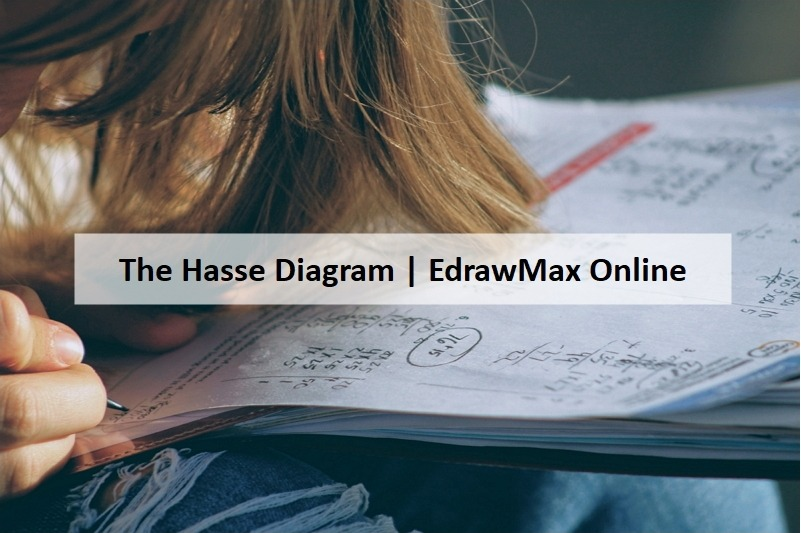#### 1.1 Relations in a Hasse Diagram

The hasse diagrams are named after Helmut Hasse. Although, he was not the first person to work on this. However, Helmut’s hasse diagrams initiated the graphical representation of POSETs. The hasse diagrams may also have binary coding of a subset (1) or not (0). They are of different shapes and sizes.

An ordinary hasse diagram or the smoothest one may have the shape of a straight line. However, a complicated diagram may have a three-dimensional shape or a 4x4 matrix. There are a few rules regarding the relationship of elements present in the hasse diagrams. These properties are:

1. Reflexivity → p ≤ p Ɐ p ꞓ B
2. Anti-symmetric → p ≤ q and q ≤ p iff p=q
3. Transitivity → if p ≤ q and q ≤ r then p ≤ r

In case of hasse diagram:

1. In the POSET, the element lesser than any other element present there is called the maximal element.
2. At the same time, the minimal element is the element that is not greater than any other elements present in the POSET.
3. The element of a POSET, which exceeds all others, is called the greatest element. Similarly, the element in the POSET that precedes the other elements is known as the least element. Both these elements in a hasse diagram are single.
4. The maximal and minimal elements in the hasse diagram can be the same, and it depends on the set it is representing.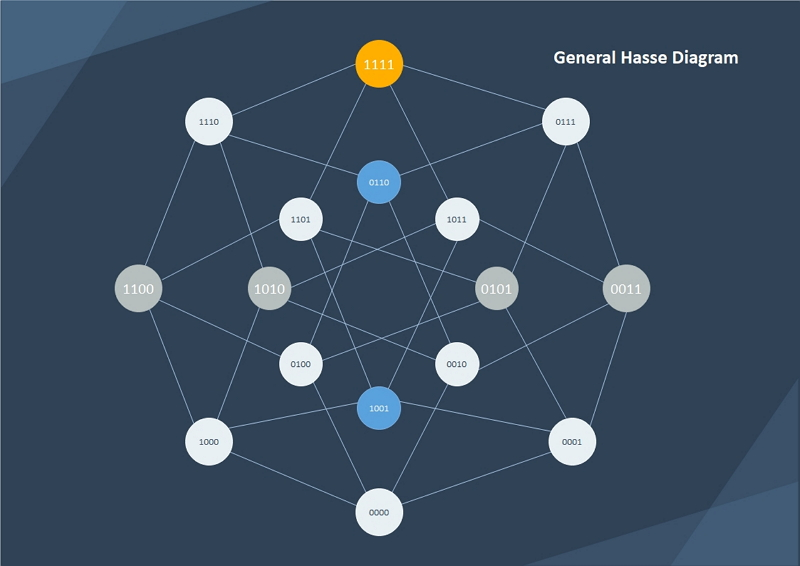Source:EdrawMax Online

## 2. How to Draw the Hasse Diagram?

The students who want to understand the ordering of sets used in it can use a hasse diagram. It is challenging to create a hasse diagram by hand.

#### 2.1 How to Create Hasse Diagram from Sketch

Here are a few steps which they can follow to draw a hasse diagram:

Step 1: The hasse diagram is also called the ordering diagram. Hence, to start with the hasse diagram, the students need to digraph the partial order.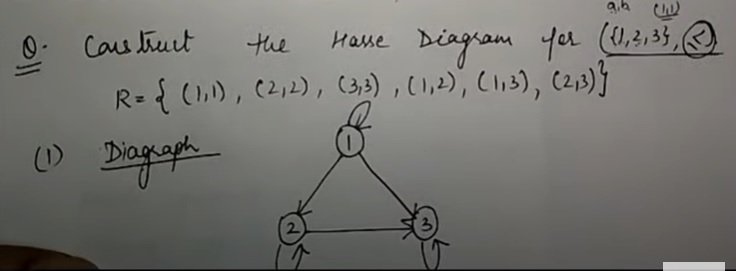Step 2: After that, they need to eliminate the self-loop present at each vertex. Then the students must also remove the elements that are present in the system due to transitivity. For example, there are three elements, 1R2, 2R3, and 1R3. After that, they have to eliminate 1R3 for transitivity.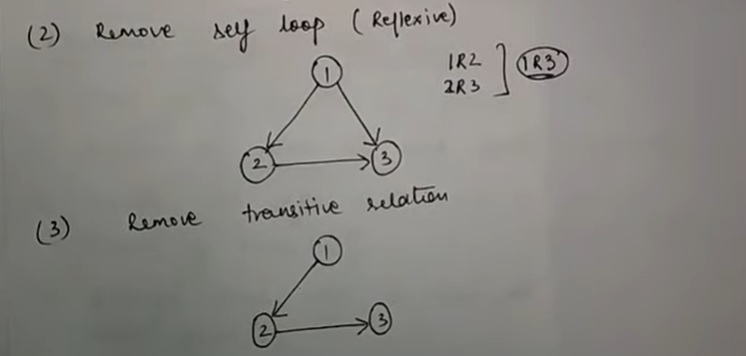Step 3: For the next part, the students have to arrange the edges so that the arrows face the upward direction. Finally, they need to erase the arrowheads and represent the nodes with dots instead of any circle or other figures.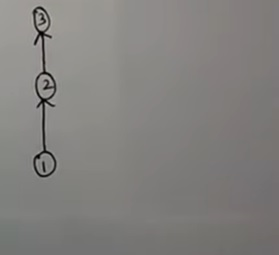#### 2.2 How to Create Hasse Diagram Online

People may get confused about the nature of it and may end up with a faulty diagram. The students need to be careful about the reflexive and transitive properties while working on their hasse diagram. To avoid this condition, the students must use the EdrawMax Online tool. The tool is user-friendly. Hence, anyone can work on it without any experience of diagramming. Its easy-to-use interface has made it a favorite diagramming tool of many users.

You can use the tool for studying multiple subjects. It comes with more than 280 different types of diagrams. Thus, today, EdrawMax has a user base of more than 25 million people. A student can create a hasse diagram with EdrawMax Online instead of drawing it by hand. Here are a few simple steps which they can follow to make a hasse diagram on the EdrawMax Online tool:

Step 1:To start with the hasse diagram, the students need to open the EdrawMax Online tool. It is a user-friendly tool. Therefore, the student can work on it without any difficulty. To continue with the process, the students need to open New. Under this ‘New’ option, the students can find the Science and Education tab.Source:EdrawMax Online

Step 2: The students can get various science and education-related videos on the tool, which they can use for their lessons. To find the hasse diagram, they need to open the Mathematics tab and choose the hasse diagram.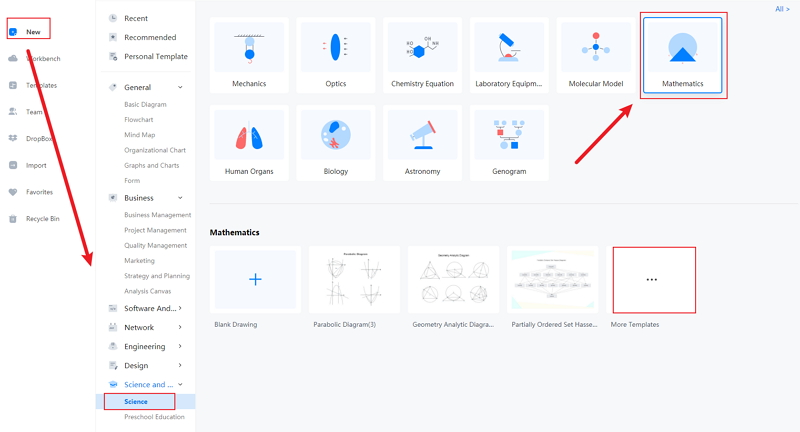Source:EdrawMax Online

Step 3: After selecting the diagram, they can edit the diagram considering the lessons they will use it. The tool has high-quality templates that require slight modification according to their need.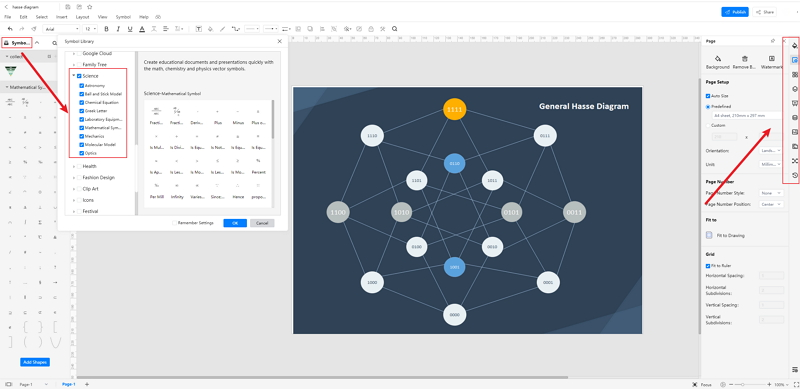Source:EdrawMax Online

Step 4: Once the student completes the modification, they can save and then export the diagram for future use. The students can save the same in multiple formats for further use in lessons, projects, and dissertation papers. They can also export the hasse diagram for future use. EdrawMax Online tool is compatible with multiple devices and operating systems. Hence, the students can smoothly work on their hasse diagram anytime and anywhere.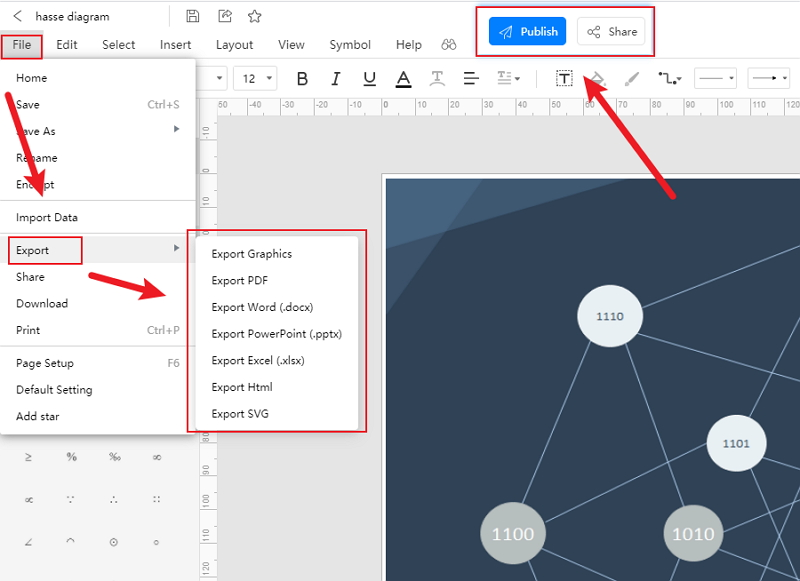Source:EdrawMax Online

## 3. Hasse Diagram Examples

The hasse diagram is an orientation of partially ordered sets. Here are a few examples of hasse diagrams:

• set A = {5, 6, 7, 8}. Let R be the relation ≤ on A. The hasse diagram of R.
• R={{5, 5}, {5, 6}, {5, 7}, {5, 8}, {6, 6}, {6, 7}, {6, 8}, {7, 7}, {7, 8}, {8, 8}}

Removed due to reflexive property: (5, 5), (6, 6), (7, 7), (8, 8).

Removed for transitive property: (5, 7), (5, 8), (6, 8).

• The second example deals with drawing the hasse diagram to find the POSET for divisibility: (4, 6, 24, 32, 48, 72). If the set is A, then:
• A = {{4, 24}, {4, 32}, {4, 48}, {4, 72}, {6, 24}, {6, 48}, {6, 72}, {24, 48}, {24, 72}}.

• Positive integer divisor of 18.
• If the set is D, then D={1, 2, 3, 6, 9, 18}, then poset A = {{1, 2}{1, 3}, {1, 6}, {1, 9}, {1, 12}, {1, 18}, {2, 6}, {2, 18}, {3, 6}, {3, 9}, {3, 18}, {6, 18}, {9, 18}}.

## 4. Conclusion

To learn about set theory and clarify their idea about partially ordered sets, the students must use their hasse diagram. It is tough to make a hasse diagram by hand, and it may be time-consuming at the same time. The students may fail to create the perfect hasse diagram for their lessons. Thus, they must use the EdrawMax Online tool, which is user-friendly. This tool can help them create a high-quality hasse diagram.

In conclusion, EdrawMax Online is a quick-start diagramming tool, which is easier to make hasse diagrams and any 280 types of diagrams. Also, it contains substantial built-in templates that you can use for free, or share your science diagrams with others in our template community.

### Related Articles